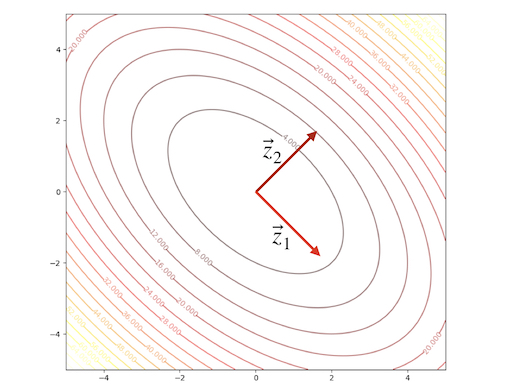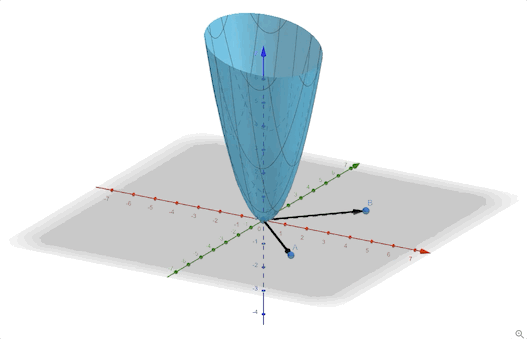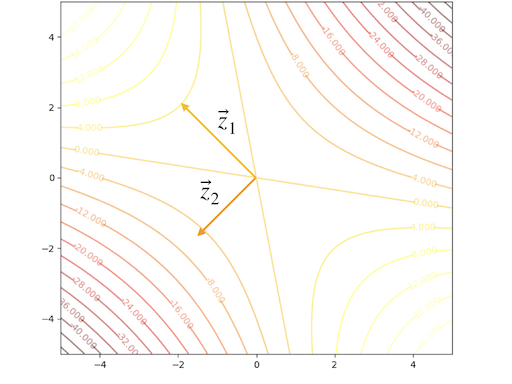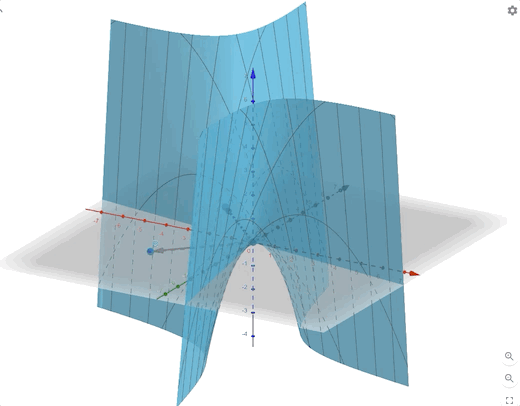## Preliminaries#

1. Linear algebra
2. Calculus 1,2
3. Taylor series

Quadratic function, a type of performance index, is universal. One of its key properties is that it can be represented in a second-order Taylor series precisely.

$F(\mathbf{x})=\frac{1}{2}\mathbf{x}^TA\mathbf{x}+\mathbf{d}\mathbf{x}+c\tag{1}$

where $$A$$ is a symmetric matrix(if it is not symmetric, it can be easily converted into symmetric). And recall the property of gradient:

$\nabla (\mathbf{h}^T\mathbf{x})=\nabla (\mathbf{x}^T\mathbf{h})=\mathbf{h}\tag{2}$

and

$\nabla (\mathbf{x}^TQ\mathbf{x})=Q\mathbf{x}+Q^T\mathbf{x}=2Q\mathbf{x}\tag{3}$

then the first order of quadratic functions are:

$\nabla F(\mathbf{x})=A\mathbf{x}+\mathbf{d}\tag{4}$

and second-order of quadratic functions are: $\nabla^2 F(\mathbf{x})=A\tag{5}$

The shape of quadratic functions can be described by eigenvalues and eigenvectors of its Hessian matrix. Hessian matrix is a symmetric matrix and its eigenvectors are mutually orthogonal, let:

$\mathbf{z}_1,\mathbf{z}_2,\cdots ,\mathbf{z}_n\tag{6}$

denote the whole set of the eigenvectors of the Hessian matrix $$A$$ and we build a matrix:

$B=\begin{bmatrix} \mathbf{z}_1&\mathbf{z}_2&\cdots &\mathbf{z}_n \end{bmatrix}\tag{7}$

and because of $$BB^T=I$$, we have $$B^{-1}=B^T$$. When the Hessian matrix is positive definite, it would have $$n$$ eigenvectors(Hessian has a full rank $$n$$) and we can change the Hessian matrix into a new matrix whose basis are the set of eigenvectors:

$A'=B^TAB=\begin{bmatrix} \lambda_1&&&\\ &\lambda_2&&\\ &&\ddots&\\ &&&\lambda_n \end{bmatrix}=\Lambda\tag{8}$

after changing the basis, the new Hessian matrix is a diagonal matrix whose elements on the diagonal are the eigenvalues. This process can be inversed because of $$BB^T=I$$:

$A=BA'B^T=B\Lambda B^T\tag{9}$

We can calculate the derivative in any directions:

$\frac{\mathbf{p}^T\nabla^2 F(\mathbf{x})\mathbf{p}}{||\mathbf{p}||^2}=\frac{\mathbf{p}^TA\mathbf{p}}{||\mathbf{p}||^2}\tag{10}$

For columns of $$B$$ can span the whole space, So we can find a vector $$\mathbf{c}$$ satisfy:

$\mathbf{p}=B\mathbf{c}\tag{11}$

then take equation(8), equation(11) into equation(10) we get:

$\frac{\mathbf{c}^TB^TAB\mathbf{c}}{\mathbf{c}^TB^TB\mathbf{c}}=\frac{\mathbf{c}^T\Lambda\mathbf{c}}{\mathbf{c}^TI\mathbf{c}}=\frac{\sum^n_{i=1}\lambda_i c_i^2}{\sum_{i=1}^n c^2_i}\tag{12}$

From equation(12), we could conclude:

$\lambda_{\text{min}}\leq \frac{\mathbf{p}^TA\mathbf{p}}{||\mathbf{p}||^2}\leq \lambda_{\text{max}}\tag{13}$

1. maximum $$2^{\text{nd}}$$ derivative occure along with $$\mathbf{z}_{\text{max}}$$(according to $$\lambda_{\text{max}}$$)
2. eigenvalues is the $$2^{\text{nd}}$$ derivative along its eigenvector.
3. eigenvectors could define a new coordinate system
4. eigenvectors are principal axes of the function contour.
5. going along with the $$\mathbf{z}_{\text{max}}$$ direction could have the largest change in function value $$|\Delta F(x)|$$
6. eigenvalues here are all positive because of positive definite.

An example:

$F(\mathbf{x})=x_1^2+x_1x_2+x_2^2 =\frac{1}{2}\mathbf{x}^T \begin{bmatrix} 2&1\\1&2 \end{bmatrix}\mathbf{x}\tag{14}$

we can calculate the eigenvectors and eigenvalues:

\begin{aligned} &\lambda_1=1&&\mathbf{z}_1=\begin{bmatrix} 1\\-1 \end{bmatrix}\\ &\lambda_2=3&&\mathbf{z}_2=\begin{bmatrix} 1\\1 \end{bmatrix} \end{aligned}\tag{15}

The contour plot and 3-D plots are:Another example:

$F(\mathbf{x})=-\frac{1}{4}x_1^2-\frac{3}{2}x_1x_2-\frac{1}{4}x_2^2 =\frac{1}{2}\mathbf{x}^T \begin{bmatrix} -0.5&-1.5\\-1.5&-0.5 \end{bmatrix}\mathbf{x}\tag{16}$

we can calculate the eigenvectors and eigenvalues:

\begin{aligned} &\lambda_1=1&&\mathbf{z}_1=\begin{bmatrix} -1\\1 \end{bmatrix}\\ &\lambda_2=-2&&\mathbf{z}_2=\begin{bmatrix} -1\\-1 \end{bmatrix} \end{aligned}\tag{17}

The contour plot and 3-D plots is:## Conclusion#

1. $$\lambda_i>0$$ or $$i=1,2,\cdots$$, $$F(x)$$ have a single strong minimum
2. $$\lambda_i<0$$ or $$i=1,2,\cdots$$, $$F(x)$$ have a single strong maximum
3. $$\lambda_i$$ have both negative and positive together. $$F(x)$$ has a saddle point
4. $$\lambda_i\geq 0$$ and have a $$\lambda_j=0$$, $$F(x)$$ has a weak minimum or has no stationary point.
5. $$\lambda_i\leq 0$$ and have a $$\lambda_j=0$$, $$F(x)$$ has a weak maximum or has no stationary point.

1. Demuth, H.B., Beale, M.H., De Jess, O. and Hagan, M.T., 2014. Neural network design. Martin Hagan.↩︎